## MP Board Class 7th Maths Solutions Chapter 12 Algebraic Expressions Ex 12.1

Question 1.
Get the algebraic expressions in the following cases using variables, constants and arithmetic operations.
(i) Subtraction of z from y.
(ii) One-half of the sum of numbers x and y.
(iii) The number z multiplied by itself.
(iv) One-fourth of the product of numbers p and Q.
(v) Numbers x and y both squared and added.
(vi) Number 5 added to three times the product of numbers m and n.
(vii) Product of numbers y and z subtracted from 10.
(viii) Sum of numbers a and b subtracted from their product.
Solution:
(i) y – z
(ii) $$\frac{1}{2}$$(x + y)
(iii) z2
(iv) $$\frac{1}{4}$$(pq)
(v) x2 + y2
(vi) 5 + 3 (mn)
(viii) ab – (a + b)

Question 2.
(i) Identify the terms and their factors in the following expressions. Show the terms and factors by tree diagrams.
(a) x – 3
(b) 1 + x + x2
(c) y – y3
(d) 5xy2 + 7x2y
(e) -ab + 2b2 – 3a2

(ii) Identify terms and factors in the expressions given below:
(a) -4x + 5
(b) -4x + 5y
(c) 5y + 3y2
(d) xy + 2x2y2
(e) pq + q
(f) 1.2ab – 2.4b + 3.6a
(g) $$\frac{3}{4}$$x + $$\frac{1}{4}$$
(h) 0.1p2 + 0.2q2
Solution:
(i)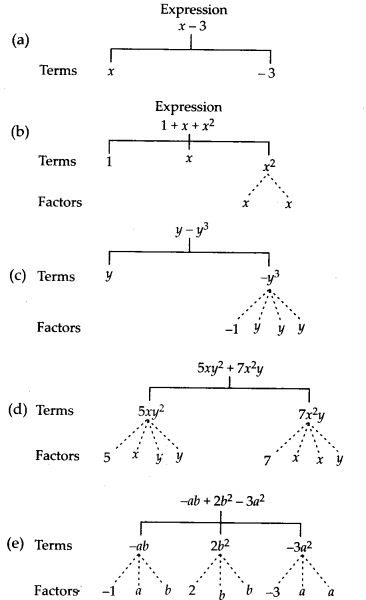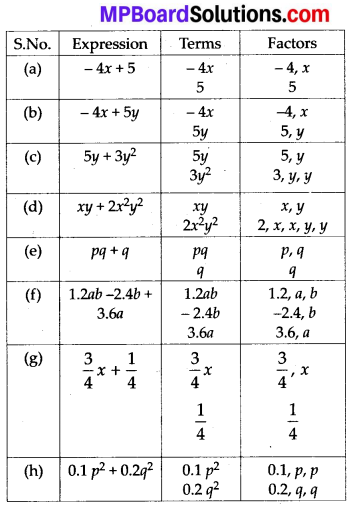Question 3.
Identify the numerical coefficients of terms (other than constants) in the following expressions:
(i) 5 – 3t2
(ii) 1 + t + t2 + t3
(iii) x + 2xy + 3y
(iv) 100m + 1000n
(v) -p2q2 + 7pq
(vi) 1.2a + 0.8b
(vii) 3.14 r2
(viii) 2(l + b)
(ix) 0.1y + 0.01y2
Solution: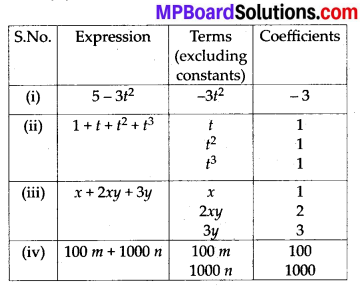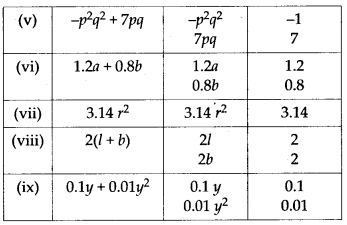Question 4.
(a) Identify terms which contains x and give the coefficient of x.
(i) y2x + y
(ii) 13y2 – 8yx
(iii) x + y + 2
(iv) 5 + z + zx
(v) 1 + x + xy
(vi) 12xy2 + 25
(vii) 7x + xy2
(b) identify terms which contains y2 and give the coefficient of y2.
(i) 8 – xy2
(ii) 5y2 + 7x
(iii) 2x2y – 15xy2 + 7y2
Solution:
(a)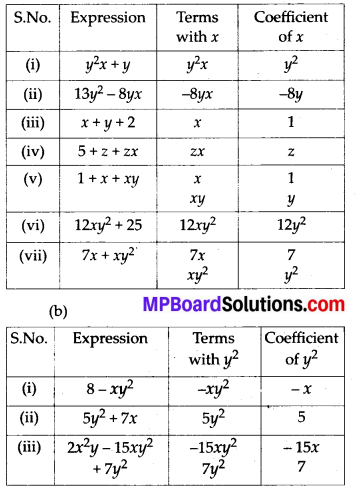Question 5.
Classify into trinomials.
(i) 4y – 7z
(ii) y2
(iii) x + y – xy
(iv) 100
(v) ab – a – b
(vi) 5 – 3t
(vii) 4p2q – 4pq2
(viii) 7mn
(ix) z2 – 3z + 8
(x) a2 + b2
(xi) z2 + z
(xii) 1 + x + x2
Solution:
The monomials, binomials and trinomials have 1, 2 and 3 unlike terms in it respectively.
(i) 4y – 7z Binomial
(ii) y2 Monomial
(iii) x + y – xy Trinomial
(iv) 100 Monomial
(v) ab – a – b Trinomial
(vi) 5 – 3t Binomial
(vii) 4p2q – 4pq2 Binomial
(viii) 7mn Monomial
(ix) z2 – 3z + 8 Trinomial
(x) a2 + b2 Binomial
(xi) z2 + z Binomial
(xii) 1 + x + x2 TrinomialQuestion 6.
State whether a given pair of terms is of like or unlike terms.
(i) 1,100
(ii) -7x, $$\frac{5}{2}$$x
(iii) -29x, -29y
(iv) 14xy, 42yx
(v) 4m2p, 4mp2
(vi) 12xz, 12x2z2
Solution:
The terms which have same algebraic factors are called like terms. However, when terms have different algebraic factors, these are called unlike terms.
(i) 1,100 Like
(ii) -7x, $$\frac{5}{2}$$x Like
(iii) -29x, -29y Unlike
(iv) 14xy, 42yx Like
(v) 4m2p, 4mp2 Unlike
(vi) 12xz, 12x2z2 Unlike

Question 7.
Identify like terms in the following:
(a) -xy2, -4yx2, 8x2, 2xy2, 7y, -11x2, -100x, -11yx, 20x2y, -6x2, y, 2xy, 3x
(b) 10pg, 7p, 8q, -p2q2, -7qp, – 100q, -23, 12q2p2, – 5p2, 41, 2405p, 78qp, 13p2g, qp2,701p2
Solution:
(a) -xy2 and 2xy2; -4yx2 and 20x2y; 8x2, -11x2 and -6x2; 7y and y, -100x and 3x; -11yx and 2xy

(b) 10pq, -7qp and 78qp; 7p and 2405p; 8q and -100y; -p2q2 and 12q2p2, -23 and 41; -5p2 and 701 p2; 13p22q and qp2